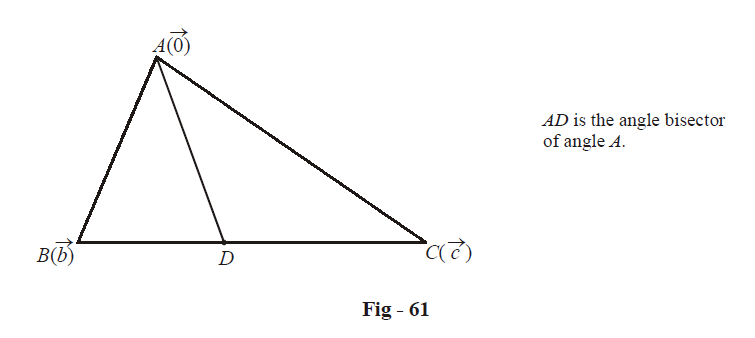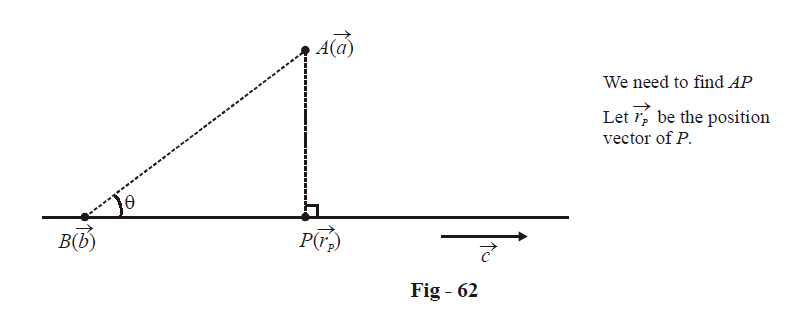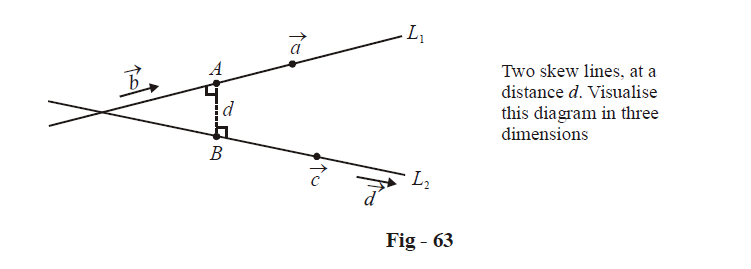# Examples On Vector Equations Of Lines Set-3

Go back to  'Vectors and 3-D Geometry'

Example – 48

Using vector methods, prove this result from plane geometry : in a triangle, the angle bisector of any angle divides the opposite side in the ratio of the sides containing the angle.

Solution: Assume a triangle ABC with position vectors of the vertices as indicated :Using the result of the previous example, the equation of AD can be written as

\begin{align}&\qquad\;\; \vec{r}=\vec{0}+\lambda \left( \hat{b}+\hat{c} \right)\;\;,\qquad\qquad\lambda \in \mathbb{R} \\ & \Rightarrow \quad \vec{r}=\lambda \left( \frac{{\vec{b}}}{\left| {\vec{b}} \right|}+\frac{{\vec{c}}}{\left| {\vec{c}} \right|} \right)\;\;,\qquad\qquad\lambda \in \mathbb{R} \\ \end{align}

Assume that D divides BC in the ratio $$\mu :1.$$ We wish to determine $$\mu .$$ The position vector of D is

$D\equiv \frac{\mu \vec{c}+\vec{b}}{\mu +1}$

Thus, for some $$\lambda ,\,\,\mu \in \mathbb{R},$$ we must have

\begin{align}&\qquad\quad \lambda \left( \frac{{\vec{b}}}{\left| {\vec{b}} \right|}+\frac{{\vec{c}}}{\left| {\vec{c}} \right|} \right)=\frac{\mu \vec{c}+\vec{b}}{\mu +1} \\\\ & \Rightarrow \quad\left( \frac{\lambda }{\left| {\vec{b}} \right|}-\frac{1}{\mu +1} \right)\vec{b}+\left( \frac{\lambda }{\left| {\vec{c}} \right|}-\frac{\mu }{\mu +1} \right)\vec{c}=\vec{0} \\ \end{align}

Since $$\vec{b}\,\,and\,\,\vec{c}$$ are non collinear, we have

\begin{align} & \frac{\lambda }{\left| {\vec{b}} \right|}=\frac{1}{\mu +1}\qquad\qquad\qquad...\left( 1 \right) \\ & \frac{\lambda }{\left| {\vec{c}} \right|}=\frac{\mu }{\mu +1}\qquad\qquad\qquad...\left( 2 \right) \\ \end{align}

Dividing (2) by (1), we have

$\mu =\frac{\left| {\vec{b}} \right|}{\left| {\vec{c}} \right|}=\frac{AB}{AC}$

Thus, D divides BC in the ratio AB : AC ; this proves the theorem.

Example – 49

Find the perpendicular distance of the point $$A(\vec{a})$$ from the line $$\vec{r}=\vec{b}+\lambda \vec{c}.$$

Solution:\begin{align}& \;AP\;=\sqrt{A{{B}^{2}}-B{{P}^{2}}} \\ \\ & \qquad=\sqrt{A{{B}^{2}}-{{(\overrightarrow{AB}\cdot \hat{C})}^{2}}} \\\\ &\qquad =\sqrt{{{\left| \vec{a}-\vec{b} \right|}^{2}}-(\vec{a}-\vec{b})\cdot \hat{c}} \\\\ &\qquad =\sqrt{{{\left| \vec{a}-\vec{b} \right|}^{2}}-\frac{(\vec{a}-\vec{b})\cdot \vec{c}}{\left| {\vec{c}} \right|}} \\ \end{align}

This is one way to specify AP. Another way could be as follows:

\begin{align}&\qquad\quad Area(\Delta ABP) = \frac{1}{2} \times BP \times AP \hfill \\\\& \Rightarrow \quad \frac{1}{2}\left| {\left( {\vec a - \vec b} \right) \times \left( {{{\vec r}_P} - \vec b} \right)} \right| = \frac{1}{2}\left| {{{\vec r}_P} - \vec b} \right|(AP) \hfill \\\\&\Rightarrow \quad \frac{1}{2}\left| {\left( {\vec a - \vec b} \right) \times \lambda \vec c} \right| = \frac{1}{2}\left| {\lambda \vec c} \right|\left( {AP} \right)&\left\{ \begin{gathered} \because {\mkern 1mu} {\mkern 1mu} {{\vec r}_P} - \vec b = \lambda \vec c \hfill \\\\ {\text{for}}{\mkern 1mu} {\text{some}}\lambda \in \mathbb{R} \hfill \\\\ \end{gathered} \right\} \hfill\\ \\& \Rightarrow \quad AP = \frac{{\left| {\left( {\vec a - \vec b} \right) \times \vec c} \right|}}{{\left| {\vec c} \right|}} \hfill \\\\&\qquad\qquad= \left| {\left( {\vec a - \vec b} \right) \times \hat c} \right| \hfill \\ \end{align}

We could have arrived at this last result even more easily:

\begin{align}&AP = AB\sin {\theta } \hfill \\\\&\quad\; = \left| {\vec a - \vec b} \right|\sin \theta \hfill \\\\&\quad\;= \left| {\,\left( {\vec a - \vec b} \right) \times \hat c\,} \right| \hfill \\ \end{align}

Example – 50

Two straight lines in space are called skew lines if they are neither parallel nor intersecting. Find the shortest distance between the two skew lines

\begin{align}& {L_1}:\vec r = \vec a + \lambda \vec b \hfill \\& {L_2}:\vec r = \vec c + \mu \vec d \hfill \\ \end{align}

Solution: First of all, convince yourself that there will be only one line along which the distance between L1 and L2 is minimum. Such a line will be perpendicular to both L1 and L2.It should be obvious that since AB is perpendicular to both L1 and L2, i.e, since AB is perpendicular to both $$\vec b$$ and $$\vec d$$it must be collinear with the vector $$\vec b \times \vec d.$$Thus, a unit vector along the direction AB is given by

$\hat n = \frac{{\vec b \times \vec d}}{{\left| {\vec b \times \vec d} \right|}}$

The distance AB is now simply the projection of the line segment joining the points $$\vec a$$ and $$\vec c$$ along the (extended) line AB. Again, visualise this in your mind; you must be very clear why this is so. We thus have,

\begin{align}& \,d = AB = \left| {\left( {\vec a - \vec c} \right) \cdot \hat n} \right| \hfill \\\\&\;\; = \left| {\frac{{\left( {\vec a - \vec c} \right) \cdot \left( {\vec b \times \vec d} \right)}}{{\left| {\vec b \times \vec d} \right|}}} \right| \hfill \\ \end{align}

We can deduce a very useful corollary from this result. The two straight lines L1 and L2 intersect (in other words, they are coplanar) if

\begin{align}&\quad\qquad d = 0 \hfill \\ & \Rightarrow \quad \left( {\vec a - \vec c} \right) \cdot \left( {\vec b \times \vec d} \right) = 0 \hfill \\& \Rightarrow \quad \vec a \cdot \left( {\vec b \times \vec d} \right) = \vec c \cdot \left( {\vec b \times \vec d} \right) \hfill \\ &\Rightarrow \quad \left[ {\vec a\,\,\,\;\;\vec b\;\;\,\,\,\vec d} \right] = \left[ {\vec c\,\,\,\;\;\vec b\;\;\,\,\,\vec d} \right] \hfill \\ \end{align}

Note that if L1 and L2 are parallel, then the distance between them can be evaluated simply as the perpendicular distance of $$\vec a$$ from L2 (or  $$\vec c$$ from L1).

Vectors
grade 11 | Questions Set 1
Vectors